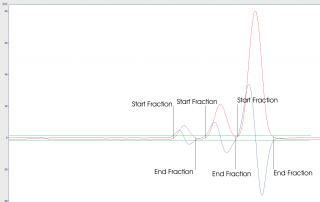# FAQ

## How does the peak detection in FC-GP work?

29.10.2015

The peak detection algorithm used in FC-GP can be explained using the figure below. The red color is the signal, the blue color is the derivative of the signal (basically a slope at that moment). The axis on the left is shown in the units of the derivative, there are two green vertical lines at 2 and -2 for illustration (let us assume the slope has a start value of 2 and an end value of 2).The first peak is collected when the derivative raises over limit 2 (first upwards black line). The peak is being collected through the peak apex (even before that the derivative falls below 2) and still being collected when the derivative falls below -2. It then starts to rise and the peak end is triggered when the derivative raises from some -4 above -2 (which is the negative of the end limit). In case the derivative on the peak end never falls below -2, the fraction will never end (until the end of the interval of the collection).